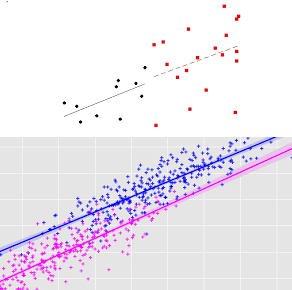Get inspired by the success stories of our students in IIT JAM MS, ISI  MStat, CMI MSc Data Science.  Learn More

# Invariant Regression Estimate | ISI MStat 2016 PSB Problem 7This cute little problem gives us the wisdom that when we minimize two functions at a single point uniquely, then their sum is also minimized at the same point. This Invariant Regression Estimate is applied to calculate the least square estimates of two group regression from ISI MStat 2016 Problem 7.

## Problem- Invariant Regression Estimate

Suppose ${(y_{i}, x_{1 i}, x_{2 i}, \ldots, x_{k i}): i=1,2, \ldots, n_{1}+n_{2}}$ represents a set of multivariate observations. It is found that the least squares linear regression fit of $y$ on $\left(x_{1}, \ldots, x_{k}\right)$ based on the first $n_{1}$ observations is the same as that based on the remaining $n_{2}$ observations, and is given by
$y=\hat{\beta}_{0}+\sum_{j=1}^{k} \hat{\beta}_{j} x_{j}$
If the regression is now performed using all $\left(n_{1}+n_{2}\right)$ observations, will the regression equation remain the same? Justify your answer.

### Prerequisites

• $f(\tilde{x})$ and $g(\tilde{x})$ are both uniquely minimized at $\tilde{x} = \tilde{x_0}$, then $f(\tilde{x}) + g(\tilde{x})$ is uniquely minimized at $\tilde{x} = \tilde{x_0}$.

## Solution

Observe that we need to find the OLS estimates of ${\beta}{i} \forall i$.

$f(\tilde{\beta}) = \sum_{i = 1}^{n_1} (y - {\beta}_{0} - (\sum_{j=1}^{k} \hat{\beta}_{j} x_{j}))^2$, where $\tilde{\beta} = ({\beta}_0, {\beta}_1, ..., {\beta}_k )$

$g(\tilde{\beta}) = \sum_{i = n_1}^{n_1+n_2} (y - {\beta}_{0} - (\sum_{j=1}^{k} \hat{\beta}_{j} x_{j}))^2$, where $\tilde{\beta} = ({\beta}_0, {\beta}_1, ..., {\beta}_k )$

$\hat{\tilde{\beta}} = (\hat{{\beta}_0}, \hat{{\beta}_1}, ..., \hat{{\beta}_k )}$

$h(\tilde{\beta}) = f(\tilde{\beta}) + g(\tilde{\beta}) = \sum_{i = 1}^{n_1+n_2} (y - {\beta}_{0} - (\sum_{j=1}^{k} \hat{\beta}_{j} x_{j}))^2$, where $\tilde{\beta} = ({\beta}_0, {\beta}_1, ..., {\beta}_k )$.

Now, $h(\tilde{\beta})$ is the loss squared erorr under the grouped regression, which needs to be minimized with respect to $\tilde{\beta}$.

Now, by the given conditions, $f(\tilde{\beta})$ and $g(\tilde{\beta})$ are both uniquely minimized at $\hat{\tilde{\beta}}$, therefore $h(\tilde{\beta}) = f(\tilde{\beta}) + g(\tilde{\beta})$ will be uniquely minimized at $\hat{\tilde{\beta}}$ by the prerequisite.

Hence, the final estimate of $\tilde{\beta}$ will be $\hat{\tilde{\beta}}$.

This cute little problem gives us the wisdom that when we minimize two functions at a single point uniquely, then their sum is also minimized at the same point. This Invariant Regression Estimate is applied to calculate the least square estimates of two group regression from ISI MStat 2016 Problem 7.

## Problem- Invariant Regression Estimate

Suppose ${(y_{i}, x_{1 i}, x_{2 i}, \ldots, x_{k i}): i=1,2, \ldots, n_{1}+n_{2}}$ represents a set of multivariate observations. It is found that the least squares linear regression fit of $y$ on $\left(x_{1}, \ldots, x_{k}\right)$ based on the first $n_{1}$ observations is the same as that based on the remaining $n_{2}$ observations, and is given by
$y=\hat{\beta}_{0}+\sum_{j=1}^{k} \hat{\beta}_{j} x_{j}$
If the regression is now performed using all $\left(n_{1}+n_{2}\right)$ observations, will the regression equation remain the same? Justify your answer.

### Prerequisites

• $f(\tilde{x})$ and $g(\tilde{x})$ are both uniquely minimized at $\tilde{x} = \tilde{x_0}$, then $f(\tilde{x}) + g(\tilde{x})$ is uniquely minimized at $\tilde{x} = \tilde{x_0}$.

## Solution

Observe that we need to find the OLS estimates of ${\beta}{i} \forall i$.

$f(\tilde{\beta}) = \sum_{i = 1}^{n_1} (y - {\beta}_{0} - (\sum_{j=1}^{k} \hat{\beta}_{j} x_{j}))^2$, where $\tilde{\beta} = ({\beta}_0, {\beta}_1, ..., {\beta}_k )$

$g(\tilde{\beta}) = \sum_{i = n_1}^{n_1+n_2} (y - {\beta}_{0} - (\sum_{j=1}^{k} \hat{\beta}_{j} x_{j}))^2$, where $\tilde{\beta} = ({\beta}_0, {\beta}_1, ..., {\beta}_k )$

$\hat{\tilde{\beta}} = (\hat{{\beta}_0}, \hat{{\beta}_1}, ..., \hat{{\beta}_k )}$

$h(\tilde{\beta}) = f(\tilde{\beta}) + g(\tilde{\beta}) = \sum_{i = 1}^{n_1+n_2} (y - {\beta}_{0} - (\sum_{j=1}^{k} \hat{\beta}_{j} x_{j}))^2$, where $\tilde{\beta} = ({\beta}_0, {\beta}_1, ..., {\beta}_k )$.

Now, $h(\tilde{\beta})$ is the loss squared erorr under the grouped regression, which needs to be minimized with respect to $\tilde{\beta}$.

Now, by the given conditions, $f(\tilde{\beta})$ and $g(\tilde{\beta})$ are both uniquely minimized at $\hat{\tilde{\beta}}$, therefore $h(\tilde{\beta}) = f(\tilde{\beta}) + g(\tilde{\beta})$ will be uniquely minimized at $\hat{\tilde{\beta}}$ by the prerequisite.

Hence, the final estimate of $\tilde{\beta}$ will be $\hat{\tilde{\beta}}$.

This site uses Akismet to reduce spam. Learn how your comment data is processed.

### 3 comments on “Invariant Regression Estimate | ISI MStat 2016 PSB Problem 7”

1.sarma klnss says:

interesting problem, probably explanation/ solution could be more elaborate, still, a very good effort

1.Srijit Mukherjee says:

Thanks for supporting us. We will try to be more elaborate. But, we will try to elaborate at such a point, when a student well equipped with the prerequisites can understand thoroughly. If someone can't then, it is an indication, that person must go back and give a quick revision.

2.priyanka submission says:

### Knowledge Partner